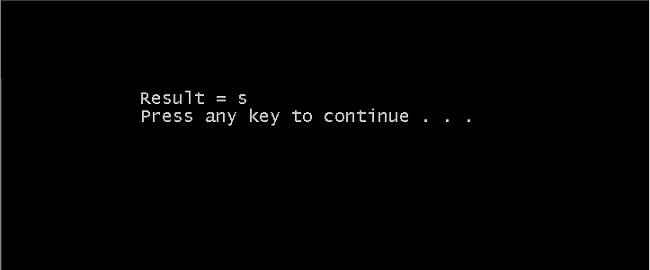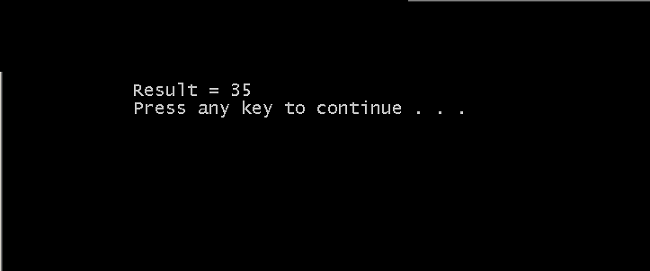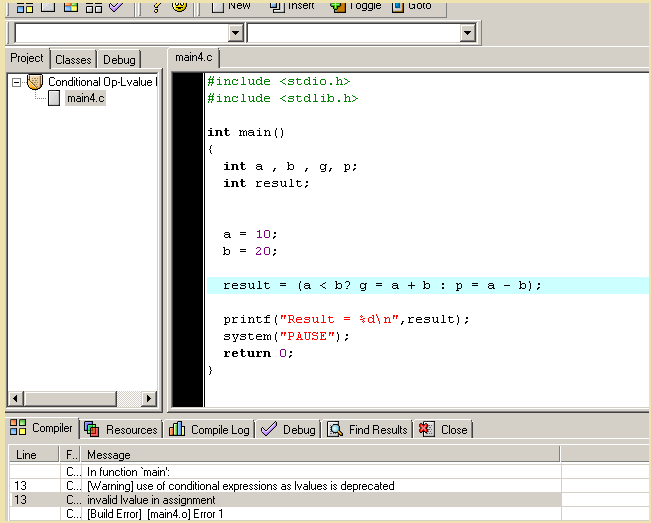## C Conditional Operators

In C programming language, sometimes it necessary to change the flow of the program.The C offers a special operator called a Conditional operator to do that.

Before you start learning about the conditional operator, be familiar with C programming basics. Skip this step if you already know the basics.

The conditional operator? : is also called the ternary operator. The syntax for the conditional operator is given below.

`(expression A ? expression B : expression C)`

## How does conditional operator work?

The expression A evaluates to true or false. If the result is true, then the expression B is executed and if the result is false, expression C is executed.

For example, consider the following code.

```int a, b, res;

a = 10;
b = 5;
res = (a >= b ? printf("%d\n", a); : printf("%d\n", b););```

The compiler checks for expression A which is “if a is greater than or equal to b”. If the expression A evaluates to true, then a value is printed.

If the expression A evaluates to false, then b value is printed as output.

## Characteristics of Conditional Operator

There are a few important characteristics of the conditional operator which are listed below. Know them will be helpful in constructing C programs using conditional operators.

1. The first expression (e.g. Expression A) must be an integral type.
2. You can nest the expressions.
3. Single expression execution.
4. Lvalue problem

### The First expression must be an integral type

The First expression must be an integral type such as integer, char, float or double. This condition makes sure that a boolean value is obtained upon execution.

Example:

For example, the following code character type is used within the conditional operator.

```#include <stdio.h>
#include <stdlib.h>

int main()
{

char a, b;
char result;
a = 'm';
b = 's';

result = (a > b? a : b);

printf("Result = %c\n",result);

system("PAUSE");
return 0;
}

```

OutputOutput-1

### You can nest the expressions

You can nest the expressions within another expression. Each expression will be executed according to its associativity. The conditional operator has right-to-left associativity.

Example:

In the code below, the conditional operator uses nested expression which is also conditional.

```#include <stdio.h>
#include <stdlib.h>

int main()
{

int a,b,c,d;
int result;
a = 10;
b = 20;

c = 5;
d = 25;

result = (a >= b? ( a <= c? (a + c) : (a - c)): (a < d? (a + d) : (a - d)));

printf("Result = %d\n",result);

system("PAUSE");

return 0;

}

```

OutputOutput-2

### Single expression execution

Single expression execution is another characteristic of the conditional operator. Only one expression is executed at a time and adding too many statements in an expression will create confusion or break the program.

For example, the nested expressions have too many statements in the above example.

So, if the conditional statement exceeds one or two expressions, then it is better to use – `if-else` structure.

### Lvalue problem

Lvalue problem is an issue that happens when both the expression B and expression C are the L-value expression. A L-value expression has a variable with memory location on left side of the equal sign (=).

For example,

```#include <stdio.h>
#include <stdlib.h>

int main()
{

int a , b , g, p;
int result;

a = 10;
b = 20;

result = (a < b? g = a + b : p = a - b);

printf("Result = %d\n",result);

system("PAUSE");

return 0;

}

```

Output:

The output of the above program is a compile-time error.Lvalue Problem – Conditional Operator